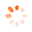Home / Products

# DESCRIPTION

Technical Description

During flow through pipes, pressure losses occur due to internal friction and friction between the fluid and the wall. When calculating pressure losses, we need to know the friction factor, a dimensionless number. The friction factor is determined with the aid of the Reynolds number, which describes the ratio of inertia forces to friction forces.

HM 150.01 enables the study of the relationship between pressure loss due to fluid friction and velocity in the pipe flow. Additionally, the pipe friction factor is determined.

The experimental unit includes a small diameter pipe section in which the laminar and turbulent flow is generated. The Reynolds number and the pipe friction factor are determined from the flow rate and pressure loss. In turbulent flow, the pipe is supplied directly from the water supply. The constant pressure at the water supply required for laminar flow is provided by a standpipe on the overflow. Valves can be used to adjust the flow rate.

The pressures in laminar flow are measured with twin tube manometers. In turbulent flow, the pressure is read on a dial-gauge manometer.

The experimental unit is positioned easily and securely on the work surface of the HM 150 base module. The water is supplied and the flow rate measured by HM 150. Alternatively, the experimental unit can be operated by the laboratory supply.

The well-structured instructional material sets out the fundamentals and provides a step-by-step guide through the experiments.

Learning Objectives / Experiments

- measurements of the pressure loss in laminar flow
- measurements of the pressure loss in turbulent flow
- determining the critical Reynolds number
- determining the pipe friction factor
- comparing the actual pipe friction factor with the theoretical friction factor

# Subscribe now